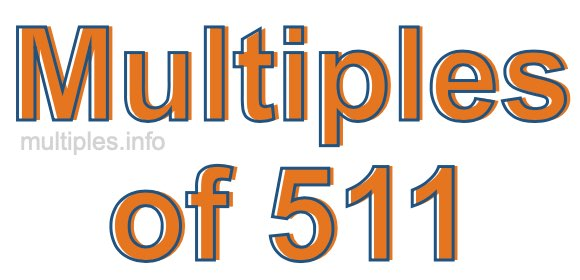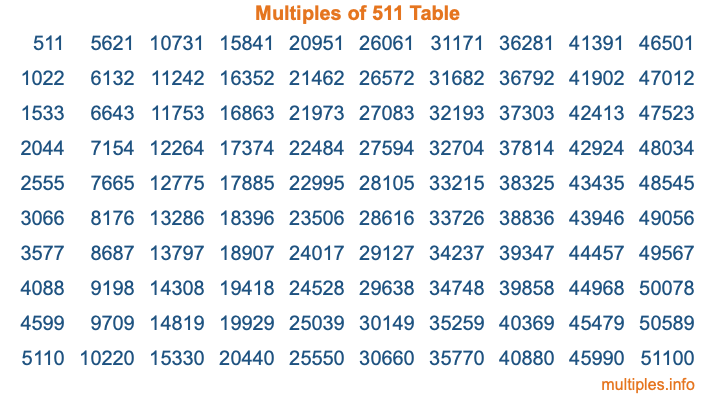Multiples of 511Welcome to the Multiples of 511 page. Here we will first teach you everything you will ever need to know about the multiples of 511, and then give you a study guide summary of everything we taught you to make sure you remember it all. Use this page to look up facts and learn information about the multiples of 511. This page will make you a multiples of five hundred eleven expert!

Definition of Multiples of 511
Multiples of 511 are all the numbers that when divided by 511 equal an integer. Each of the multiples of 511 are called a multiple. A multiple of 511 is created by multiplying 511 by an integer.

Therefore, to create a list of multiples of 511, you start with 1 multiplied by 511, then 2 multiplied by 511, then 3 multiplied by 511, and so on for as long as you want. Thus, the list of the first five multiples of 511 is 511, 1022, 1533, 2044, and 2555. To see a larger list of multiples of 511, see the printable image of Multiples of 511 further down on this page. We also have a category where you can choose any nth multiple of 511.

Multiples of 511 Checker
The Multiples of 511 Checker below checks to see if any number of your choice is a multiple of 511. In other words, it checks to see if there is any number (integer) that when multiplied by 511 will equal your number. To do that, we divide your number by 511. If the the quotient is an integer, then your number is a multiple of 511.

Is  a multiple of 511?

Least Common Multiple of 511 and ...
A Least Common Multiple (LCM) is the lowest multiple that two or more numbers have in common. This is also called the smallest common multiple or lowest common multiple and is useful to know when you are adding our subtracting fractions. Enter one or more numbers below (511 is already entered) to find the LCM.

Check out our LCM Calculator if you need more details about the Least Common Multiple or if you need the LCM for different numbers for adding and subtraction fractions.

nth Multiple of 511
As we stated above, 511 is the first multiple of 511, 1022 is the second multiple of 511, 1533 is the third multiple of 511, and so on. Enter a number below to find the nth multiple of 511.

th multiple of 511

Multiples of 511 vs Factors of 511
511 is a multiple of 511 and a factor of 511, but that is where the similarities end. All postive multiples of 511 are 511 or greater than 511. All positive factors of 511 are 511 or less than 511.

Below is the beginning list of multiples of 511 and the factors of 511 so you can compare:

Multiples of 511: 511, 1022, 1533, 2044, 2555, etc.

Factors of 511: 1, 7, 73, 511

As you can see, the multiples of 511 are all the numbers that you can divide by 511 to get a whole number. The factors of 511, on the other hand, are all the whole numbers that you can multiply by another whole number to get 511.

It's also interesting to note that if a number (x) is a factor of 511, then 511 will also be a multiple of that number (x).

Multiples of 511 vs Divisors of 511
The divisors of 511 are all the integers that 511 can be divided by evenly. Below is a list of the divisors of 511.

Divisors of 511: 1, 7, 73, 511

The interesting thing to note here is that if you take any multiple of 511 and divide it by a divisor of 511, you will see that the quotient is an integer.

Multiples of 511 Table
Below is an image of the first 100 multiples of 511 in a table. The table is in chronological order, column by column. The first column has the first ten multiples of 511, the second column has the next ten multiples of 511, and so on.The Multiples of 511 Table is also referred to as the 511 Times Table or Times Table of 511. You are welcome to print out our table for your studies.

Negative Multiples of 511
Although not often discussed or needed in math, it is worth mentioning that you can make a list of negative multiples of 511 by multiplying 511 by -1, then by -2, then by -3, and so on, to get the following list of negative multiples of 511:

-511, -1022, -1533, -2044, -2555, etc.

Multiples of 511 Summary
Below is a summary of important Multiples of 511 facts that we have discussed on this page. To retain the knowledge on this page, we recommend that you read through the summary and explain to yourself or a study partner why they hold true.

There are an infinite number of multiples of 511.

A multiple of 511 divided by 511 will equal a whole number.

511 divided by a factor of 511 equals a divisor of 511.

The nth multiple of 511 is n times 511.

The largest factor of 511 is equal to the first positive multiple of 511.

511 is a multiple of every factor of 511.

511 is a multiple of 511.

A multiple of 511 divided by a divisor of 511 equals an integer.

511 divided by a divisor of 511 equals a factor of 511.

Any integer times 511 will equal a multiple of 511.

Multiples of a Number
Here you can get the multiples of another number, all with the same attention to detail as we did for multiples of 511 on this page.

Multiples of
Multiples of 512
Did you find our page about multiples of five hundred eleven educational? Do you want more knowledge? Check out the multiples of the next number on our list!

Copyright  |   Privacy Policy  |   Disclaimer  |   Contact